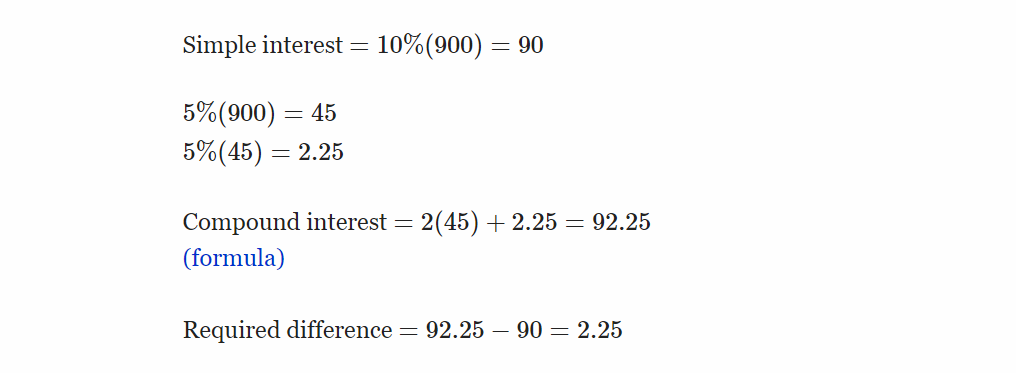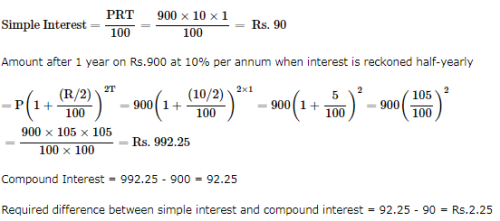Courses

The difference between simple interest and compound on Rs. 900 for one year at 10% per annum reckoned half-yearly is: a)Rs. 3b)Rs. 2.25c)Rs. 4.5d)Rs. 4Correct answer is option 'B'. Can you explain this answer?

Related TestJitender Pal Sep 06, 2021
Answer :b)Rs. 2.25Mrinmoy Kundu Oct 01, 2018N H Oct 26, 2021
P=90
year=1
rate=10

simple insterst=pnr÷100
900*1*10÷100=90

compound
p=90
half yearly=365÷2
rate=10

A=p(1+R÷100)n

900×(105)2
900×105×105÷200=992.25

992.5-900=2.25

compound=2.25
simple insterst=

Top Courses for CATRelated ContentCompound InterestVideo | 14:58 minSimple Interest & Compound InterestVideo | 14:49 minSimple Interest and Compound InterestDoc | 5 PagesExample - Simple Interest & Compound InterestVideo | 14:49 minSimple Interest vs Compound InterestVideo | 11:14 min
Do you know? How Toppers prepare for CAT Exam
With help of the best CAT teachers & toppers, We have prepared a guide for student who are preparing for CAT : 15 Steps to clear CAT Exam